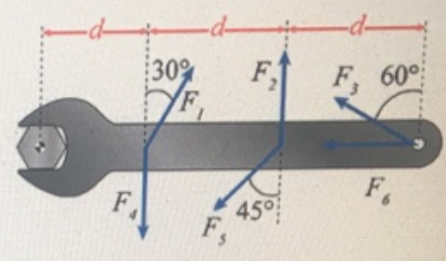# Problem: Problem 6: A wrench is used to apply a torque to a bolt. Forces F, through F6 all have the same magnitude. The point of application of force F, (and F) is a distance d from the center of rotation of the bolt. The application points of F, F2, and F3 are all distance d apart, as shown. Use the convention that counter-clockwise torques are positive; clockwise torques are negative.Part (a) Which of the labeled forces will apply the largest magnitude torque on the bolt?Part (b) Which of the labeled forces will apply the smallest magnitude torque on the bolt?

###### FREE Expert Solution

Torque:

$\overline{){\mathbf{\tau }}{\mathbf{=}}{\mathbf{r}}{\mathbf{F}}{\mathbf{s}}{\mathbf{i}}{\mathbf{n}}{\mathbf{\theta }}}$

where r is the position vector and θ is the angle between the force vector and the lower arm vector.

Part (a)

From the equation for calculating torque, we see that torque is directly proportional to sinθ.

Therefore, the larger the value of sinθ the larger the torque.

84% (318 ratings)###### Problem Details

Problem 6: A wrench is used to apply a torque to a bolt. Forces F, through F6 all have the same magnitude. The point of application of force F, (and F) is a distance d from the center of rotation of the bolt. The application points of F, F2, and F3 are all distance d apart, as shown. Use the convention that counter-clockwise torques are positive; clockwise torques are negative.Part (a) Which of the labeled forces will apply the largest magnitude torque on the bolt?

Part (b) Which of the labeled forces will apply the smallest magnitude torque on the bolt?